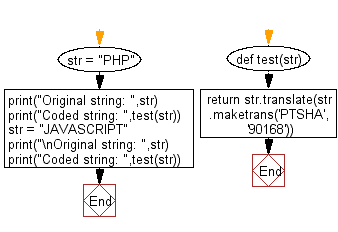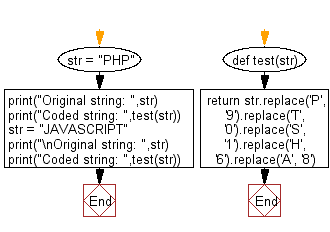﻿ Python: Create a coded string from a given string, using specified formula - w3resource

# Python: Create a coded string from a given string, using specified formula

## Python Basic - 1: Exercise-121 with Solution

Write a Python program to create a coded string from a given string, using a specified formula.

Replace all 'P' with '9', 'T' with '0', 'S' with '1', 'H' with '6' and 'A' with '8'

Sample Solution-1:

Python Code:

``````def test(str):
return str.translate(str.maketrans('PTSHA', '90168'))
str = "PHP"
print("Original string: ",str)
print("Coded string: ",test(str))
str = "JAVASCRIPT"
print("\nOriginal string: ",str)
print("Coded string: ",test(str))
``````

Sample Output:

```Original string:  PHP
Coded string:  969

Original string:  JAVASCRIPT
Coded string:  J8V81CRI90
```

Flowchart:Sample Solution-2:

Python Code:

``````def test(str):
return str.replace('P', '9').replace('T', '0').replace('S', '1').replace('H', '6').replace('A', '8')

str = "PHP"
print("Original string: ",str)
print("Coded string: ",test(str))
str = "JAVASCRIPT"
print("\nOriginal string: ",str)
print("Coded string: ",test(str))
``````

Sample Output:

```Original string:  PHP
Coded string:  969

Original string:  JAVASCRIPT
Coded string:  J8V81CRI90
```

Flowchart:Python Code Editor:

Have another way to solve this solution? Contribute your code (and comments) through Disqus.

What is the difficulty level of this exercise?

Test your Programming skills with w3resource's quiz.

﻿